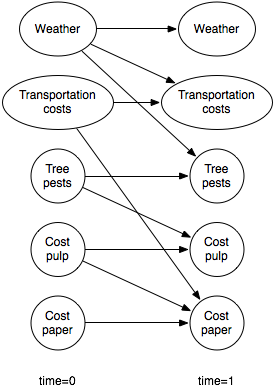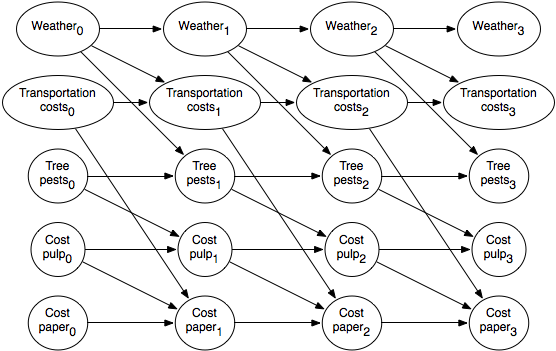6.5.4 Dynamic Belief Networks

You do not have to represent the state at a particular time as a single variable. It is often more natural to represent the state in terms of features.

A dynamic belief network (DBN) is a belief network with regular repeated structure. It is like a (hidden) Markov model, but the states and the observations are represented in terms of features. Assume that time is discrete. If F is a feature, we write Ft as the random variable that represented the value of variable F at time t. A dynamic belief network makes the following assumptions:

• The set of features is the same at each time.
• For any time t>0, the parents of variable Ft are variables at time t or time t-1, such that the graph for any time is acyclic. The structure does not depend on the value of t (except t=0 is a special case).
• The conditional probability distribution of how each variable depends on its parents is the same for every time t>0.

Thus, a dynamic belief network specifies a belief network for time t=0, and for each variable Ft specifies P(Ft|parents(Ft)), where the parents of Ft are in the same or previous time step. This is specified for t as a free parameter; the conditional probabilities can be used for any time t>0. As in a belief network, directed cycles are not allowed.

The model for a dynamic belief network can be represented as a two-step belief network that represents the variables at the first two times (times 0 and 1). That is, for each feature F there are two variables, F0 and F1; parents(F0) only include variables for time 0, and parents(F1) can be variables at time 0 or 1, as long as the resulting graph is acyclic. Associated with the network are the probabilities P(F0|parents(F0)) and P(F1|parents(F1)). Because of the repeated structure, P(Fi|parents(Fi)), for i>1, has exactly the same structure and the same conditional probability values as P(F1|parents(F1)).

Example 6.31: Suppose the trading agent wants to model the dynamics of the price of a commodity such as printer paper. To represent this domain, the designer models what variables affect the price and the other variables. Suppose the cost of pulp and the transportation costs directly affect the price of paper. The transportation costs are affected by the weather. The pulp cost is affected by the prevalence of tree pests, which in turn depend on the weather. Suppose that each variable depends on the values of the previous time step. A two-stage dynamic belief network representing these dependencies is shown in Figure 6.19.Figure 6.19: Two-stage dynamic belief network for paper pricing

Note that, in this figure, the variables are initially independent.

This two-stage dynamic belief network can be expanded into a regular dynamic belief network by replicating the nodes for each time step, and the parents for future steps are a copy of the parents for the time 1 variables.Figure 6.20: Expanded dynamic belief network for paper pricing

An expanded belief network is shown in Figure 6.20. The subscripts represent the time that the variable is referring to.